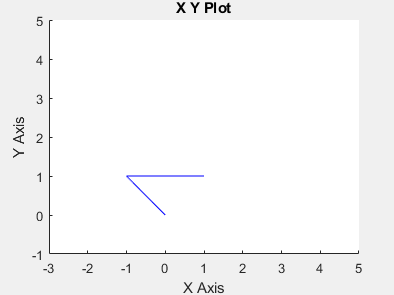# Generate Trapezoidal Velocity Trajectory

This example shows how to generate a trapezoidal velocity trajectory using the Trapezoidal Velocity block.

Open the model. The waypoints are specified in the block mask. The position and velocity outputs are connect to scopes and the position is plotted to an XY Plot. The Time input is just a ramp signal to simulate time progressing.

`open_system('trapvel_traj_ex1.slx')`Run the Simulation. The first figure shows the output of the `q` vector for the positions of the trajectory. The second figure shows the `qdd` vector for the velocity. Notice the trapezoidal profile for each waypoint transition. The XY Plot shows the actual 2-D trajectory, which hits the specified waypoints.

PositionsVelocities